Understanding Super-Convergence

In this blog post, we will take a look at the Super-Convergence research paper and try to understand:

• The meaning and benefits of super-convergence
• Practical considerations when using super-convergence
• How to use super-convergence with the fast.ai library

Introduction

Neural networks are trained using an algorithm called stochastic gradient descent (SGD). SGD is an iterative method that calculates the direction in which we need to update the parameters of the network so that its performance improves. We then update the parameters of the network accordingly.

When updating the parameters, we use a parameter called the learning rate to control the size of the steps we take when updating the parameters during SGD. A higher learning rate results in larger updates to a network's parameters.

Higher learning rates allow us to train networks faster. However, since we start with randomly initialized weights, if we start training with a high learning rate then our neural network will not learn. Also, if we end training with a high learning rate then there is a chance that our network won't settle at a minima.

So we want to start and end training neural networks with a low learning rate, but use a high learning rate in between. Leslie Smith proposes a method that behaves just like this in this paper, and calls it the 1cycle training policy.

The 1cycle training policy

In the 1cycle training policy, we start training a neural network with a very small learning rate. We increase the learning rate linearly with each batch until it reaches a maximum value (this is called the warmup phase). We then decrease the learning rate until it reaches the initial learning rate value (this is called the annealing phase). The paper then advises us to continue training the model for a few more epochs while reducing the learning from the value we started with to one several orders of magnitude lower.

If we were to plot the learning rate we use to train the network against training iterations, we would get something like this: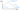Benefits of super-convergence

Using the 1cycle policy allows training with much higher learning rates than usual. As a result, neural networks tend to train much faster than usual. This is what Leslie Smith refers to as super-convergence in the paper.

Also, using high learning rates allows the network to bypass local minima and move towards smoother parts of the loss function. This results in lesser overfitting.

A great explanation for this can be found in chapter 13 of fastbook:

... a model that generalizes well is one whose loss would not change very much if you changed the input by a small amount. If a model trains at a large learning rate for quite a while, and can find a good loss when doing so, it must have found an area that also generalizes well, because it is jumping around a lot from batch to batch. -- Deep Learning for Coders with fastai & PyTorch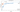This comparison of test accuracies with super-convergence (in orange) and without it (in blue) shows how it allows us to train networks faster and with a higher test accuracy.

According to the paper, this boost in performance due to super-convergence is even more prominent when the amount of training data is limited.

The regularizing effect of super-convergence

Super-convergence is a combination of the 1cycle training policy and a high maximum learning rate. Using a high learning rate has a regularizing effect and we therefore need to reduce other forms of regularization such as batch sizes, weight decay and dropout.

The paper specifically mentions that super-convergence was slightly more effective with larger batch sizes, since smaller batch sizes also have a regularizing effect (they are the "stochastic" in stochastic gradient descent).

Checking if super-convergence is possible

The LR range test introduced by Leslie Smith in Cyclical Learning Rates for Training Neural Networks can be used to determine if super-convergence is possible for a given neural network architecture.

In the LR range test, we start training with a very small learning rate and linearly increase it up to a very large value and plot the loss or accuracy against the learning rate. Typically, there is a distinct peak in such a graph since the network converges with a low learning rate, and finally starts diverging as the learning rate becomes too large: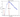However, with certain architectures even when a very large learning rate is reached in the LR range test, the test accuracy remains consistently high: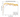Network architectures that exhibit this kind of behavior have the potential of benefiting most from super-convergence.

The 1cycle policy in fastai

Using the 1cycle training policy in fastai is extremely simple!

Instances of Learner have a fit_one_cycle method that allows you to train a network using the 1cycle policy.

You can specify the maximum learning rate as a parameter to this method.

learn = Learner(...)
# Train for 10 epochs with a maximum learning rate of 0.06
learn.fit_one_cycle(10, 0.06)

Yes, it's that simple!

The 1cycle policy implemented in fastai is slightly different from the one described in the paper. Instead of linearly increasing and then linearly decreasing the learning rate, fastai uses an approach called cosine annealing: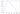Conclusion

We have looked at what super-convergence is and the benefits it brings. In particular, the ability to train networks faster and with a higher test accuracy, especially if the amount of labeled data for training is limited.

We also saw how to use the 1cycle training policy with a high maximum learning rate to achieve super-convergence in theory, and how the use the policy practically using the fast.ai library.

We also saw that since using a high learning rate has a regularizing effect, we need to minimize other forms of regularization we use.